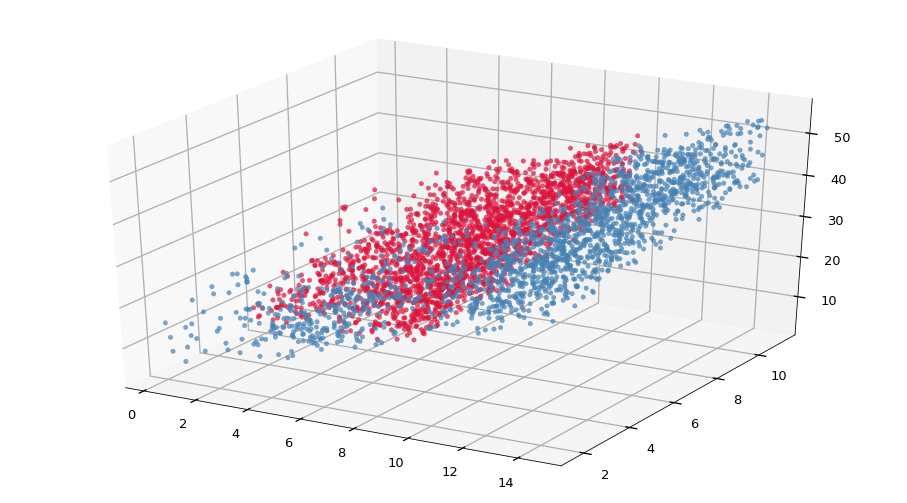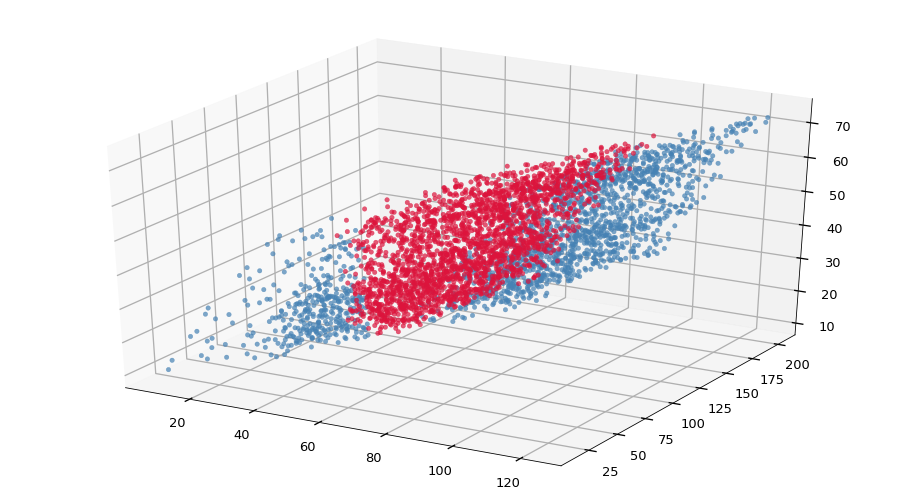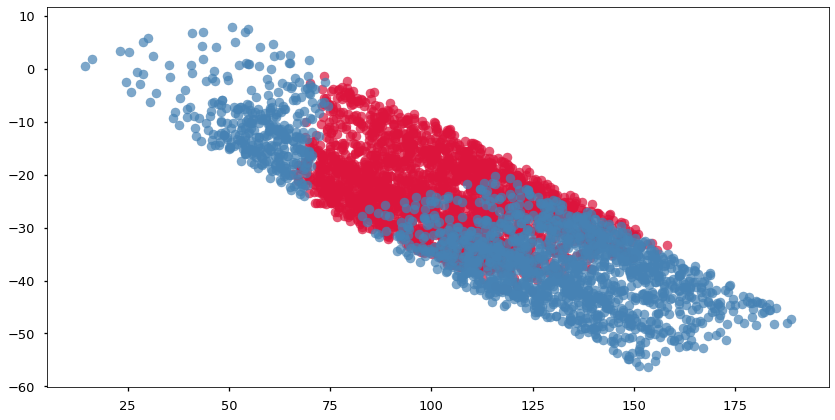# 08.01 Principal Component Analysis¶

PCA is an unsupervised algorithm which reduces the feature space. The algorithm builds a projection of the feature space into a space with a smaller number of dimensions. The new feature space is not necessarily meaningful in terms of the original features, because the projection plane (or hyperplane) may cross the features diagonally.

Let us start with the idea of projections. Data points are no more than multidimensional arrays, and in Linear Algebra multiplication between arrays of different dimensions performs a projection between the dimensions. We import a handful of things and build a simple example of a function in $3$ dimensions.

In :
import numpy as np
import pandas as pd
import matplotlib.pyplot as plt
%matplotlib inline
plt.style.use('seaborn-talk')
from mpl_toolkits import mplot3d


We will have two classes: red and blue. These data will reside in three dimensions, and we could call the class itself a fourth dimension. The classes have no meaning in this case but they allow us to visualize which side of the data we are looking at.

The meshgrid produces all combinations between $x$ and $y$ features, and we build a almost-dependent $z$ feature. The $z$ feature will be a function of $x$ and $y$ but with some noise added in. We have.

$$z = f(x, y) \approx x^2 + y$$

And for the classes, we define them in terms of $x$ and $z$

$$red = z > 20, x < 10 \approx \sqrt{20 - y} < x < 10$$

The full set look as follows.

In :
points = 64
x = np.linspace(0, 10, points) + 7*np.random.rand(points)
y = np.linspace(0, 10, points) + 3*np.random.rand(points)
gx, gy = np.meshgrid(x, y)
z = 2*gx + gy + 15*np.random.rand(points, points)
red = (z > 20) & (x < 10)

fig = plt.figure(figsize=(16, 9))
ax = fig.add_subplot(111, projection='3d')
ax.scatter(gx[red], gy[red], z[red], c='crimson', alpha=0.7)
ax.scatter(gx[~red], gy[~red], z[~red], c='steelblue', alpha=0.7);The combination of the arrays is three dimensional but a s we saw before we can melt the three dimensional representation into a two dimensional one. We build a matrix (a two dimensional array or a data frame) representing each feature as a column. This is the most common data representation we see.

In :
df = pd.DataFrame({'x': gx.reshape(-1), 'y': gy.reshape(-1), 'z': z.reshape(-1)})
df

Out:
x y z
0 0.325168 2.718422 12.430248
1 3.928762 2.718422 20.879095
2 4.412740 2.718422 12.547498
3 6.080664 2.718422 15.690980
4 2.462841 2.718422 18.423135
... ... ... ...
4091 13.801802 10.036509 43.700409
4092 14.407722 10.036509 41.457652
4093 12.937877 10.036509 42.865677
4094 12.218582 10.036509 45.049026
4095 13.288020 10.036509 51.157064

4096 rows × 3 columns

Now that we have all our data as a matrix we can do linear algebra on top of it. Matrix multiplication is only possible if the left operand has the same number of columns as the right operand has of rows.

$$X_{n \times m} T_{p \times q}$$

Is only possible if $m = p$. In other words $A_{2 \times 3}$ can multiply $B_{3 \times 5}$ but $B_{3 \times 5}$ cannot multiply $A_{2 \times 3}$. Yes, you did read that right, matrix multiplication is not commutative. $A_{2 \times 3}$ must be on the left hand side of the multiplication and $B_{3 \times 5}$ must be on the right hand side. And the result of the multiplication will have $n$ rows and $q$ columns.

$$X_{n \times m} T_{p \times q} = M_{n \times q}$$

Our piece of data is a matrix which we will call $X_{4096 \times 3}$. We can (left) multiply a matrix $T_{n \times 4096}$ by our matrix, or we can (right) multiply our matrix by a matrix $T_{3 \times m}$. The terms left multiplication and right multiplication are from linear algebra and mean the use of the other matrix by which we multiply on the left or right of the matrix we want to multiply.

Since $T_{3 \times m}$ is a lot easier to build than $T_{n \times 4096}$, we will build the former and left multiply our data by it. For simplicity we will use a square matrix $T_{3 \times 3}$. We use the letter $T$ for this matrix to mean transform, and we write TR in the code. Note that @ is Python's own matrix multiplication operator.

In :
TR = np.array([
[1, 2, -1],
[0, 1,  3],
[2, 3,  1],
])
M = df.values @ TR
mx, my, mz = M[:, 0], M[:, 1], M[:, 2]

mred = red.reshape(-1)
fig = plt.figure(figsize=(16, 9))
ax = fig.add_subplot(111, projection='3d')
ax.scatter(mx[mred], my[mred], mz[mred], c='crimson', alpha=0.7)
ax.scatter(mx[~mred], my[~mred], mz[~mred], c='steelblue', alpha=0.7);Since we multiplied across the features of our $X_{4096 \times 3}$ we kept the number of samples ($4096$) and since the matrix $T_{3 \times 3}$ is square we kept the number of features (dimensions). The end results is that the values in side $T_{3 \times 3}$ move, rotate or stretch our data; but the end result is still $4096$ points in three dimensional space.

The multiplication we are interested in though is to reduce the number of the dimensions. Since our $X_{4096 \times 3}$ has $3$ columns then our $MT{3 \times m}$ must have $3$ rows in order for the multiplication to be possible (defined). But the number of columns of $T_{3 \times m}$ is not required to be $3$.

If we right multiply our data, $X_{4096 \times 3}$ by a matrix $T_{3 \times 2}$ the result will be some matrix $M_{4096 \times 2}$. This $M_{4096 \times 2}$ is a representation of $4096$ points in $2$ dimensions, hence we projected our our data points from $3$ dimensions into $2$ dimensions.

In :
TR = np.array([
[1, -1],
[1,  2],
[3, -1],
])
M = df.values @ TR
mx, my = M[:, 0], M[:, 1]

mred = red.reshape(-1)
fig, ax = plt.subplots(figsize=(14, 7))
ax.scatter(mx[mred], my[mred], c='crimson', alpha=0.7)
ax.scatter(mx[~mred], my[~mred], c='steelblue', alpha=0.7);We now know know how to project data between dimensions. All we need to do is to create a transform matrix ($T$) with the same number of rows as the number of columns (dimensions, features) in our data and multiply them. In other words, we know how to perform a rather simple dimensionality reduction.

The problem is that the numbers inside the matrix $T$ shape the projection in different ways. The numbers squeeze, rotate, and move the data around. Moreover, before we had $4096 \cdot 3 = 12288$ values representing our data now we have $4096 \cdot 2 = 8192$ values. Therefore we lost some information about our data in the process of the projection. If we want to minimize the amount of information we lose during the projection we must have a way of selecting the best numbers for the values inside $T$. Enters Principal Component Analysis (PCA).

PCA find the best projection that minimizes the loss of variance within the data we project. For the time being let us argue that PCA has a way of finding the best possible transformation matrix to minimize the loss in variance across the data. Another detail of the transformation matrix is that the rotation operation that the transformation performs is always around the origin. Hence PCA will move the data so that the mean in every dimension is zero, i.e. that the data is centered at the origin. This way the rotations happen around the middle of the data itself.

When used from sklearn, PCA behaves just as any other sklearn model. PCA expects to be given the number of components we project onto, this is a hyperparameter of the PCA algorithm. We are rather confident that we can take the data above and live with its variance in two dimensions only, therefore we will use $2$ components.

Since the $z$ feature is almost fully dependent on the values of $x$ and $y$, the variance in $z$ can be explained by the variance in $x$ and $y$. We expect that PCA will figure that out and flatten out the $z$ feature. PCA is a transformation hence we use the transform method (or more exactly fit_transform here) to project the data. The result is still the same data but in less dimensions. This can be visualized or passed on to other models.

In :
from sklearn.decomposition import PCA

X = np.c_[gx.reshape(-1), gy.reshape(-1), z.reshape(-1)]
pca = PCA(n_components=2)
X_new = pca.fit_transform(X)
red_new = red.reshape(-1)

fig, ax = plt.subplots(figsize=(14, 7))
ax.scatter(X_new[red_new, 0], X_new[red_new, 1], color='crimson', alpha=0.7)
ax.scatter(X_new[~red_new, 0], X_new[~red_new, 1], color='steelblue', alpha=0.7)
pca.components_.T

Out:
array([[-0.30114245, -0.48460744],
[-0.11265113,  0.87328861],
[-0.94690176,  0.05022583]])